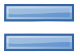Showing posts with label Object class. Show all posts
Showing posts with label Object class. Show all posts## Difference between Object class equals() and String class equals()

In this tutorial we will see about what is the difference between Object class equals() and String class equals() method. Before discussing on difference on both methods lets see about both classes. We all knows that Object class is the base class for all the classes the Java. Totally 12 methods are there in Object class and each methods can be Overridden while we implement our
own class on necessary.
Lets see on how equals() method implemented in both the classes.

Object Class:

equals() method in Object class used to compare whether some other Object is "equal to" this one. It compares only the Object level are same in both the sides. And also we need to note that it is necessary to override hashCode() method whenever equals() method is overridden, as to maintain the general contract for the hashCode method, which states that same objects must have equal hash codes.
It will return only of both the Objects are same else it will return false.

String Class:

Basically equals() method in String class is overridden from Object class and used to compare whether some other Object is "equal to" this one, along with also Objects will be typecast to String and each character wise comparison will be made in equals() method.
First it will check for both the Objects are same and in case if both the Objects are same it will return true else true if the given object represents a String equivalent to this string, false otherwise.

Lets see code of both the equals() method in Object class and String class.

equals() method in Object class:

```public boolean equals(Object obj) {
return (this == obj);
}
```

equals() method in String class:

```public boolean equals(Object anObject) {
if (this == anObject) {
return true;
}
if (anObject instanceof String) {
String anotherString = (String) anObject;
int n = count;
if (n == anotherString.count) {
char v1[] = value;
char v2[] = anotherString.value;
int i = offset;
int j = anotherString.offset;
while (n-- != 0) {
if (v1[i++] != v2[j++])
return false;
}
return true;
}
}
return false;
}
```

As we discussed above whenever equals() method overridden then we need to override hashCode() method also to maintain the general contract for the hashCode method. In that case String class also need to Override hashCode() method, lets see the hashCode() method which present in the String class.

hashCode() method in String class:
```
```
```
```
```public int hashCode() {
int h = hash;
if (h == 0) {
int off = offset;
char val[] = value;
int len = count;
for (int i = 0; i < len; i++) {
h = 31 * h + val[off++];
}
hash = h;
}
return h;
}
```